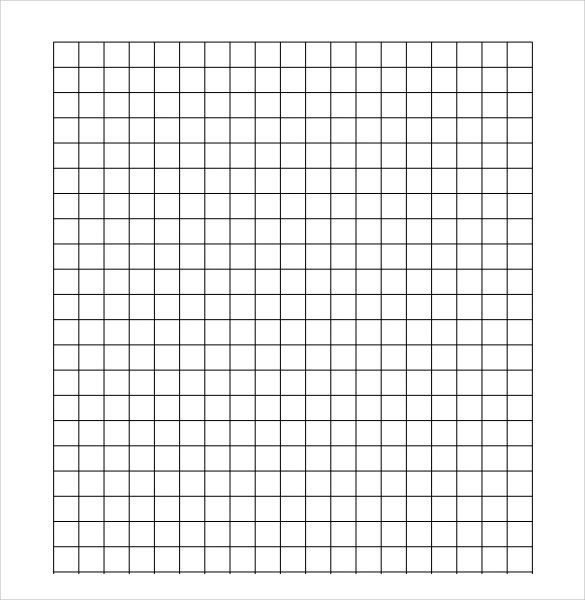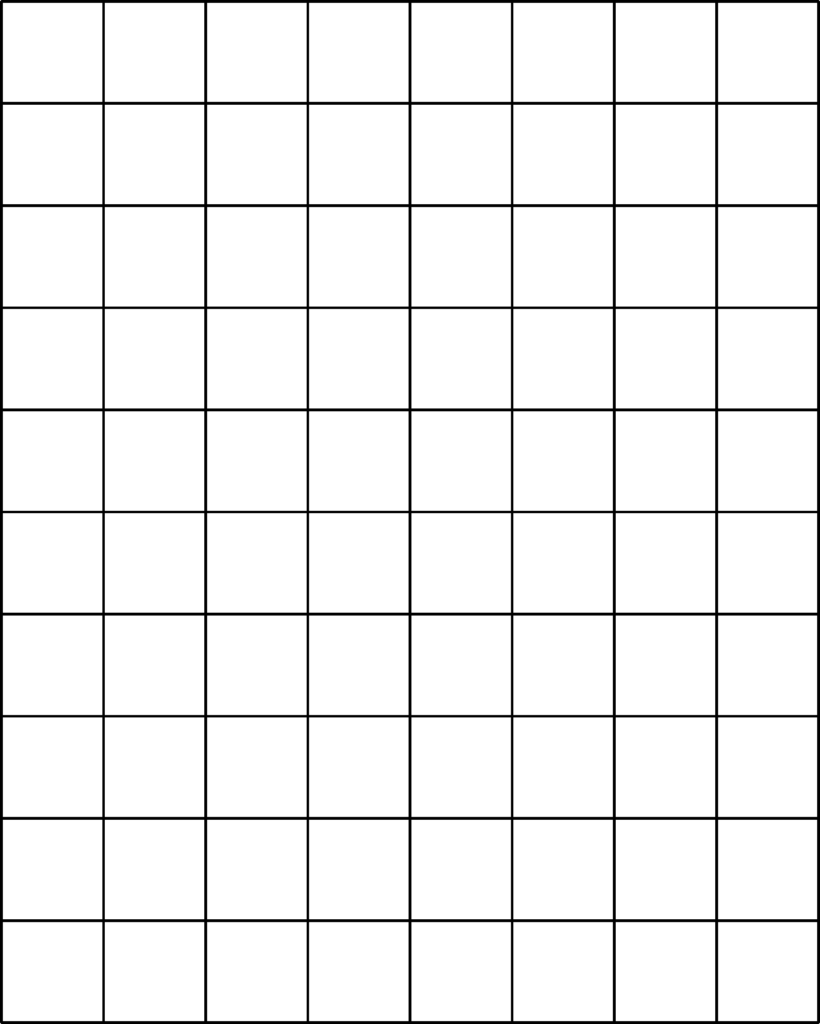# Different Sizes of Graph Paper – CM, MM, Inches

Graph paper is the most commonly used paper by math, engineering, and art students. Different sizes of graph papers are available and through this post, we are covering them. If you are here to know about the different sizes of graph papers then read the information below. As a student, you must be aware of the graph paper types because only then will you be able to select the correct type depending on the type of work you are involved in. Different work requires different graph paper, therefore, one must be aware of the type of graph paper.

Contents

## Different Sizes of Graph Paper

Graph paper is also known as coordinate paper, grid paper, or squared paper. It is a writing paper that is printed with fine lines making up a regular grid. The lines are often used as guides for plotting graphs of functions or experimental data and drawing curves. You can see the use of graph papers in the domain of mathematics and engineering.

Below you can see different sizes of graph papers with a brief introduction and a free template to download in PDF format.

### Centimeter Graph Paper

Centimeter Graph Paper is commonly used in the domain of mathematics. Kids use this type of graph paper for learning math concepts like calculus or geometry. It’s also used for line plotting, charting, or even design. Below you can see a graph paper template that is available for free download in a printable format. Check out the centimeter Grid paper below and download it for your use.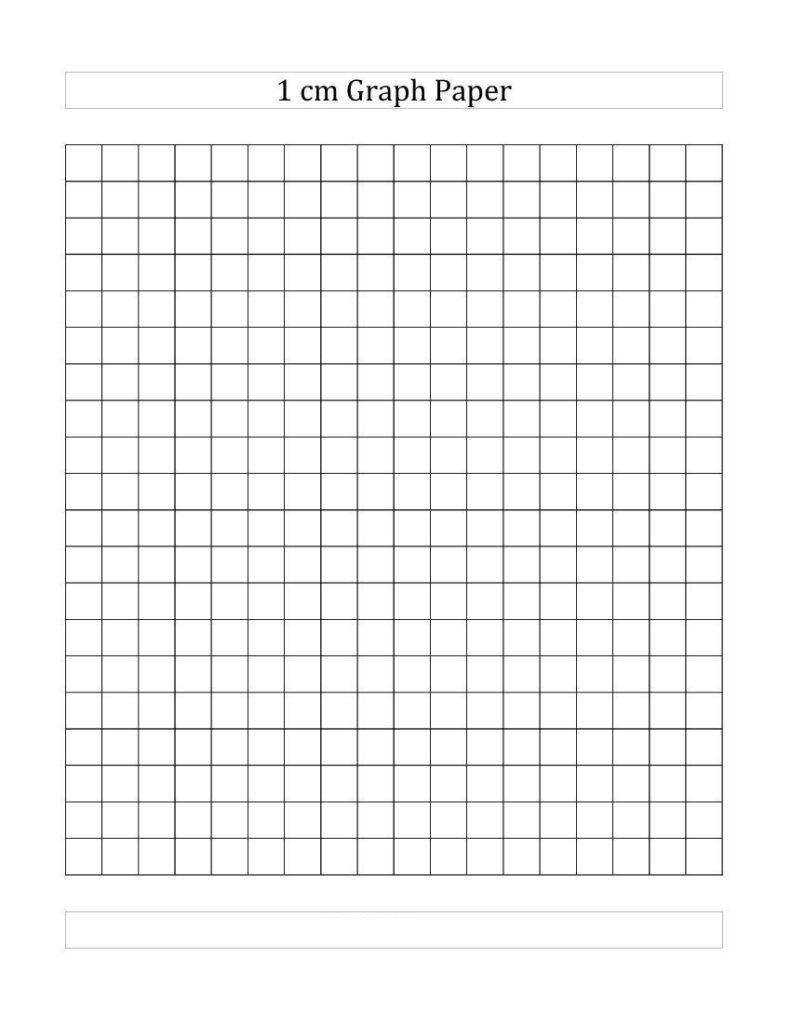### 5 MM Graph Paper

As you can see there are horizontal and vertical lines on graph paper and they are at a distance of 5mm from each other. You can see the use of this graph paper in the field of engineering. If you are studying or working in the field of engineering then do check out the graphing paper below, download it, take a print-out for your use, and start using it.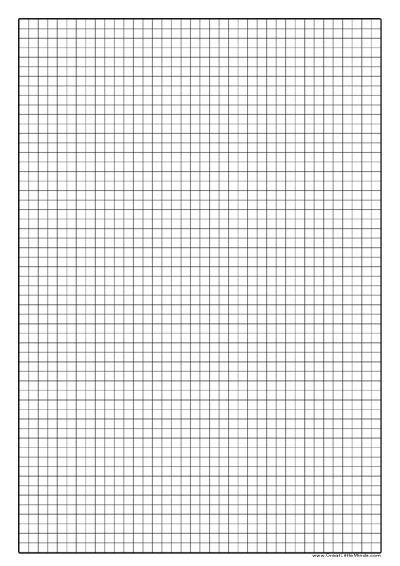### 10 Squares Per Inch of Graph Paper

If you are working with inches then check out the 10 squares per inch graphing paper below to manage your work with precision. Below you can see a graph paper template in the portrait orientation. Download this graphing paper for free download in a printable format.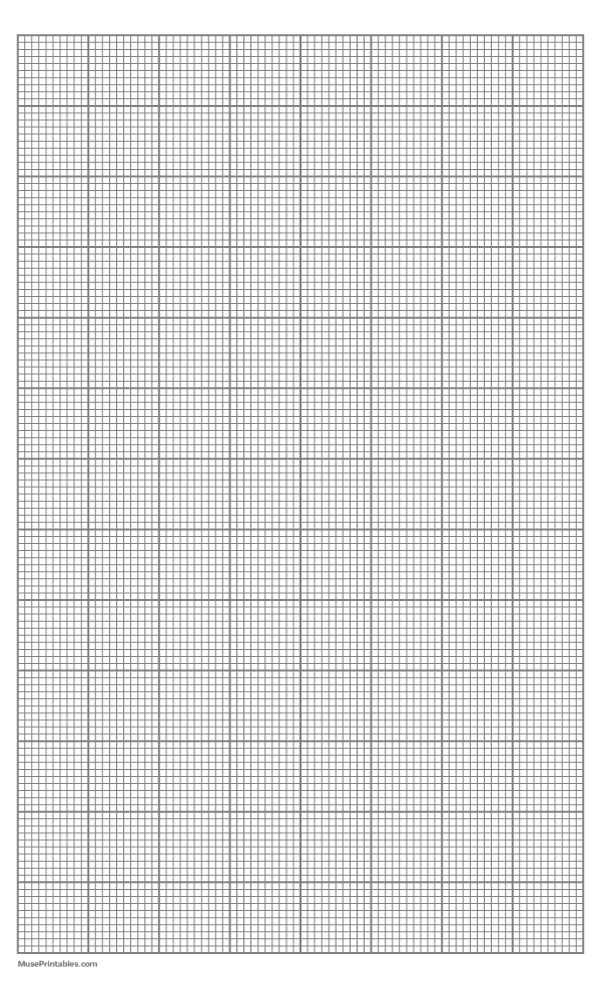### 1/4 Inches Graph Paper

You can see the use of 1/4 Inches Graph Paper in schools. Kids often use this type of graph paper to learn the concept of mathematics such as addition, subtraction, etc. Apart from that, this graphing paper can also be used by engineering and art students for their work needs. You can also use this graphing paper if you want to use it for technical drawing. Check out the paper below in portrait form and download it for your use.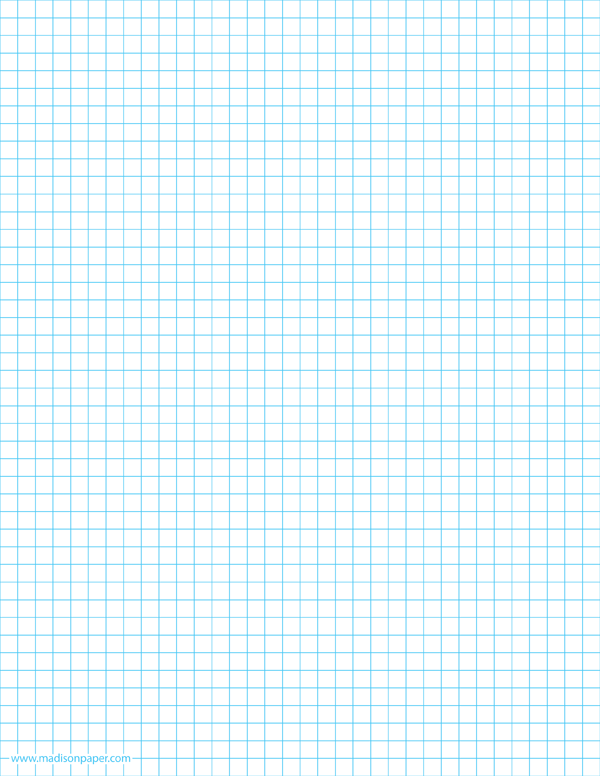### 1/2 Half Inch Graph Paper

Check out the printable 1/2 Half Inch Graph Paper below. This type of graph paper is commonly used in the field of mathematics, people use them for keeping bullet journals, and sketching purposes. As you can see this graphing paper has small square grids that are half an inch in size. If you need graph paper for studying or work purposes, then make sure to have a template downloaded with you because you never know when you are going to require this graph paper.